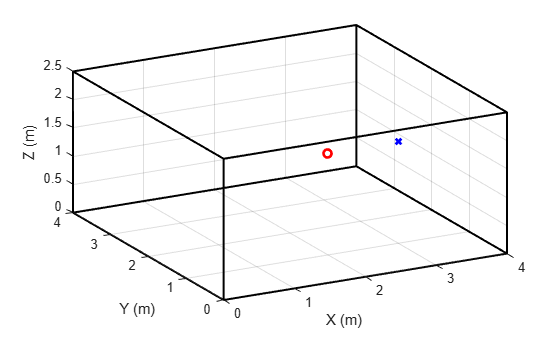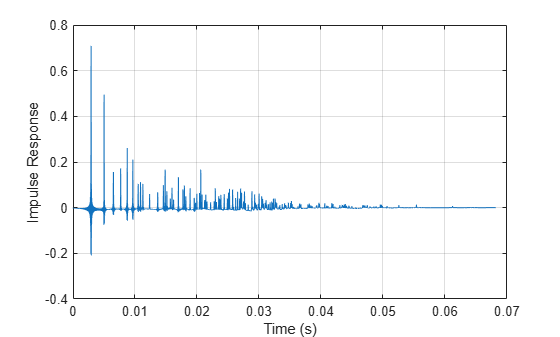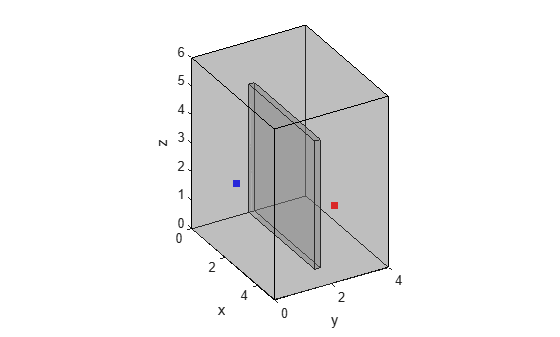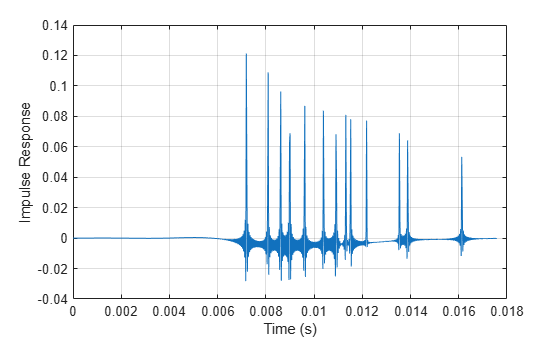# Room Impulse Response Simulation with the Image-Source Method and HRTF Interpolation

Room impulse response simulation aims to model the reverberant properties of a space without having to perform acoustic measurements. Many geometric and wave-based room acoustic simulation methods exist in the literature . The image-source method is a popular and relatively straightforward geometric method . It models the specular reflections between a transmitter and a receiver.

This example showcases the image-source method for a simple "shoebox" (cuboid) room. The example also uses head-related transfer function (HRTF) interpolation to simulate the received sound at the ears of the listener.

### Define Room Parameters

You simulate the impulse response of a shoebox empty room.

Define the room dimensions, in meters (width, length and height, respectively).

`roomDimensions = [4 4 2.5];`

You treat the receiver and transmitter as points within the space of the room. Define their coordinates, in meters.

```receiverCoord = [2 1 1.8]; sourceCoord = [3 1 1.8];```

Plot the room space along with the receiver (red circle) and transmitter (blue x).

```h = figure; plotRoom(roomDimensions,receiverCoord,sourceCoord,h)```### The Image-Source Method

Image-source is a geometric simulation method that models specular sound reflection paths between the source and receiver. It assumes that sound travels in straight lines (rays) which undergo perfect reflections when they encounter an obstacle (in our case, one of the four walls, the floor, or the ceiling of the room).

When a sound ray hits a wall, it spawns a mirrored "image" source. The image source is the symmetrical reflection of the original source with respect to the encountered boundary. Higher-order reflections (rays that reach the receiver after bouncing off multiple obstacles) are modeled by repeating the mirroring process with respect to each encountered obstacle.

As an example, consider the ray that bounces off two walls and the floor before arriving at the receiver. Define the coordinates of the equivalent image for this ray.

`imageSource = [-sourceCoord(1) -sourceCoord(2) -sourceCoord(3)];`

The ray is modeled by the straight line connecting the image to the receiver. The length of this straight line is equal to the traveled distance from the original source to the receiver along the reflected ray.

Visualize the image-source and the resulting path.

```plot3(imageSource(1),imageSource(2),imageSource(3),"gx",LineWidth=2) plot3([imageSource(1) receiverCoord(1)], ... [imageSource(2) receiverCoord(2)], ... [imageSource(3) receiverCoord(3)],Color="k",LineWidth=2)```### Visualize Multiple Images

To calculate the room impulse response, add the contributions of a large number of source images.

Extend the visible space around the room to ensure the images appear in the plot.

```h2 = figure; plotRoom(roomDimensions,receiverCoord,sourceCoord,h2) Lx = roomDimensions(1); Ly = roomDimensions(2); Lz = roomDimensions(3); xlim([-3*Lx,3*Lx]); ylim([-3*Ly,3*Ly]); zlim([-3*Lz,3*Lz]);```Visualize a subset of the source images. Compute the image coordinates based on equations 6 and 7 in .

Model the eight combinations stemming from possible relflections along the x-, y- and z- axes.

```x = sourceCoord(1); y = sourceCoord(2); z = sourceCoord(3); sourceXYZ = [-x -y -z;... -x -y z;... -x y -z;... -x y z;... x -y -z;... x -y z;... x y -z;... x y z].';```

Model scenarios with multiple reflections by looping over the x-, y- and z- axes. These loops have infinite ranges in theory. You will see how to practically limit the ranges in the next section. For now, select arbitrary limits for the loops.

```% Increase the range to plot more images nVect = -2:2; lVect = -2:2; mVect = -2:2; for n = nVect for l = lVect for m = mVect xyz = [n*2*Lx; l*2*Ly; m*2*Lz]; isourceCoords = xyz - sourceXYZ; for kk=1:8 isourceCoord=isourceCoords(:,kk); plot3(isourceCoord(1),isourceCoord(2),isourceCoord(3),"g*") end end end end```### Restricting the Number of Simulated Images

The number of images is theoretically infinite. Restrict the number of images by limiting the computed impulse response length to the time by which the reverberated sound pressure drops below a certain level. Here, you use the reverberation time RT60 , which is the time by which the sound level has dropped by 60 dB.

You use Sabine's formula to calculate RT60.

#### Define Wall Absorption Coefficients

First, define the absorption coefficients of the walls. The absorption coefficient is a measure of how much sound is absorbed (rather than reflected) when hitting a surface.

The absorption coefficients are frequency-dependent, and are defined at the frequencies defined in the variable `FVect` .

```FVect = [125 250 500 1000 2000 4000]; A = [0.10 0.20 0.40 0.60 0.50 0.60;... 0.10 0.20 0.40 0.60 0.50 0.60;... 0.10 0.20 0.40 0.60 0.50 0.60;... 0.10 0.20 0.40 0.60 0.50 0.60;... 0.02 0.03 0.03 0.03 0.04 0.07;... 0.02 0.03 0.03 0.03 0.04 0.07].';```

#### Estimate RT60

Compute RT60 based on Sabine's formula.

First, compute the room's volume.

`V = Lx*Ly*Lz;`

Next, compute the total wall surface area of the room.

```WallXZ = Lx*Lz; WallYZ = Ly*Lz; WallXY = Lx*Ly;```

Compute the frequency-dependent effective absorbing area of the room surfaces.

`S = WallYZ*(A(:,1)+A(:,2))+WallXZ.*(A(:,3)+A(:,4))+WallXY.*(A(:,5)+A(:,6));`

Compute the frequency-dependent RT60, in seconds, based on Sabine's equation. Notice that RT60 is frequency-dependent: There are 6 different RT60 values, one for each frequency band.

```c = 343; % Speed of sound (m/s) RT60 = (55.25/c)*V./S```
```RT60 = 6×1 1.3886 0.7191 0.3799 0.2581 0.3028 0.2455 ```

Deduce the maximum impulse response length (in samples) based on the largest value in RT60. Assume a sample rate of 48 kHz.

```fs = 48000; impResLength = fix(max(RT60)*fs)```
```impResLength = 66653 ```

Express the maximum range of the impulse response in meters.

`impResRange=c*(1/fs)*impResLength`
```impResRange = 476.2912 ```

Use this value to limit the range over which to compute images. In this example, to limit the run time, you restrict the loop ranges to [-10;10].

```nMax = min(ceil(impResRange./(2.*Lx)),10); lMax = min(ceil(impResRange./(2.*Ly)),10); mMax = min(ceil(impResRange./(2.*Lz)),10);```

### Derive Contribution of One Image

In this section, you derive the contribution of one image to the room impulse response.

You later obtain the full room impulse response by summing the contributions of all images under consideration.

Derive the pressure reflection coefficients from the absorption coefficients.

`B=sqrt(1-A);`

Store the reflection coefficients for each wall in a separate variable.

```BX1=B(:,1); BX2=B(:,2); BY1=B(:,3); BY2=B(:,4); BZ1=B(:,5); BZ2=B(:,6);```

Model the eight permutations representing the absence or presence of reflection on the x-, y-, and z- axes.

```surface_coeff=[0 0 0; 0 0 1; 0 1 0; 0 1 1; 1 0 0; 1 0 1; 1 1 0; 1 1 1]; q = surface_coeff(:,1).'; j = surface_coeff(:,2).'; k = surface_coeff(:,3).'; ```

In this section, you focus on the contribution of a single image. Select index values corresponding to an arbitrary image.

```n = 1; l = 1; m = 1; p = 1;```

#### Compute Image Delay

The contribution of each image is defined by two values:

• Delay: The time it takes the signal to reach the receiver.

• Power: The (frequency-dependent) energy level of the signal when it reaches the receiver.

You start by computing the image delay. The delay is related to the total distance traveled by the wave from the image to the receiver.

Get the coordinates of the image.

`isourceCoord = [n*2*Lx; l*2*Ly; m*2*Lz] - sourceXYZ(:,p);`

Calculate the delay (in samples) at which the contribution occurs.

```dist = norm((isourceCoord(:)-receiverCoord(:)),2); delay = (fs/c).*dist;```

#### Compute Image Power

Now compute the frequency-dependent magnitude of the contribution.

`ImagePower = BX1.^abs(n-q(p)).*BY1.^abs(l-j(p)).*BZ1.^abs(m-k(p)).*BX2.^abs(n).*(BY2.^abs(l)).*(BZ2.^abs(m));`

#### Derive Image Contribution

The image power is only defined at 6 frequencies. Here, you first interpolate the response to the entire frequency (Nyquist) range, and then perform an inverse FFT operation to derive the image's contribution to the impulse response.

Extend the energy level to incorporate zero and Nyquist frequencies.

```FVect2=[0 FVect fs/2]'; ImagePower2 = [ImagePower(1); ImagePower(:); ImagePower(6)];```

In this example, use an FFT length of 512.

```FFTLength = 512; HalfLength = fix(FFTLength./2); OneSidedLength = HalfLength+1;```

Interpolate the response to the entire frequency range.

`ImagePower2 = interp1(FVect2./(fs/2),ImagePower2,linspace(0,1,257)).';`

Convert the response to two-sided.

`ImagePower2 = [ImagePower2; conj(ImagePower2(HalfLength:-1:2))];`

Convert the frequency response to the time-based contribution of the image.

`h_ImagePower = real(ifft(ImagePower2,FFTLength));`

Smooth the response by applying a Hann window.

```win = hann(FFTLength+1); h_ImagePower = win.*[h_ImagePower(OneSidedLength:FFTLength); ... h_ImagePower(1:OneSidedLength)];```

### HRTF Modeling

You have derived the image's contribution to the impulse response, where you assumed that the receiver is a point in space. Here, you derive the image's contribution at the ears of a listener located at the receiver coordinates by using 3-D head-related transfer function (HRTF) interpolation.

You use the ARI HRTF data set . Load the data set.

`ARIDataset = load("ReferenceHRTF.mat");`

Express the `hrtfData `as an array of size (Number of source measurements) × 2 × (Sample lengths).

```hrtfData = permute(ARIDataset.hrtfData,[2 3 1]); sourcePosition = ARIDataset.sourcePosition(:,[1 2]);```Calculate the elevation and azimuth corresponding to the coordinates of the image source.

```sensor_xyz=receiverCoord; xyz=isourceCoord-sensor_xyz; hyp = sqrt(xyz(1)^2+xyz(2)^2); elevation = atan(xyz(3)./(hyp+eps)); azimuth = atan2(xyz(2),xyz(1));```

The desired HRTF position is formed by the computed elevation and azimuth.

`desiredPosition = [azimuth elevation]*180/pi;`

Calculate the HRTF at the desired position.

```interpolatedIR = interpolateHRTF(hrtfData,sourcePosition,desiredPosition); interpolatedIR = squeeze(permute(interpolatedIR,[3 2 1]));```

Incorporate the HRTF into the response using convolution.

```interpolatedIR = [interpolatedIR; zeros(512,2)]; h = []; h(:,1) = filter(h_ImagePower,1,interpolatedIR(:,1)); h(:,2) = filter(h_ImagePower,1,interpolatedIR(:,2)); ```

Plot the overall contribution of the selected image. This contribution is added to the overall impulse response at the computed image delay.

```figure plot(1:size(h,1),h) grid on xlabel("Sample Index") ylabel("Impulse Response") legend("Left","Right")```### Compute The Impulse Response

In this section, you compute the overall impulse response by summing the contributions of individual images. The contribution of each image is computed exactly like in the previous section.

The helper function `HelperImageSource` encapsulates the steps you went over in the previous section. It computes the impulse response by summing image contributions.

```useHRTF = true; h = HelperImageSource(roomDimensions,receiverCoord,sourceCoord,A,FVect,fs,useHRTF,hrtfData,sourcePosition);```
```Starting parallel pool (parpool) using the 'local' profile ... Connected to the parallel pool (number of workers: 4). ```

### Visualize Impulse Response

Plot the impulse response.

```figure t= (1/fs)*(0:size(h,1)-1); plot(t,h) grid on xlabel("Time (s)") ylabel("Impulse Response") legend("Left","Right")```### Auralization

Apply the impulse response to an audio signal.

```[audioIn,fs] = audioread("FunkyDrums-44p1-stereo-25secs.mp3"); audioIn = audioIn(:,1);```

Simulate the received audio by filtering with the impulse response.

```y1 = filter(h(:,1),1,audioIn); y2 = filter(h(:,2),1,audioIn); y = [y1 y2];```

Listen to a few seconds of the original audio.

```T = 10; sound(audioIn(1:fs*T),fs) pause(T)```

Listen to a few seconds of the received audio.

`sound(y(1:fs*T),fs);`

### References

 "Overview of geometrical room acoustic modeling techniques", Lauri Savioja, Journal of the Acoustical Society of America 138, 708 (2015).

 Allen, J and Berkley, D. "Image Method for efficiently simulating small‐room acoustics", The Journal of the Acoustical Society of America, Vol 65, No.4, pp. 943‐950, 1978.

### Helper Functions

```function plotRoom(roomDimensions,receiverCoord,sourceCoord,figHandle) % PLOTROOM Plot room, transmitter and receiver figure(figHandle) X = [0;roomDimensions(1);roomDimensions(1);0;0]; Y = [0;0;roomDimensions(2);roomDimensions(2);0]; Z = [0;0;0;0;0]; figure; hold on; plot3(X,Y,Z,"k","LineWidth",1.5); % draw a square in the xy plane with z = 0 plot3(X,Y,Z+roomDimensions(3),"k","LineWidth",1.5); % draw a square in the xy plane with z = 1 set(gca,"View",[-28,35]); % set the azimuth and elevation of the plot for k=1:length(X)-1 plot3([X(k);X(k)],[Y(k);Y(k)],[0;roomDimensions(3)],"k","LineWidth",1.5); end grid on xlabel("X (m)") ylabel("Y (m)") zlabel("Z (m)") plot3(sourceCoord(1),sourceCoord(2),sourceCoord(3),"bx","LineWidth",2) plot3(receiverCoord(1),receiverCoord(2),receiverCoord(3),"ro","LineWidth",2) end function h = HelperImageSource(roomDimensions,receiverCoord, .... sourceCoord,A,FVect,fs,useHRTF,varargin) % HELPERIMAGESOURCE Estimate impulse response of shoebox room % roomDimensions: Room dimensions, specified as a row vector with three % values. % receiverCoord: Receiver coordinates, specified as a row vector with 3 % values % sourceCoord: Source coordinates, specified as a row vector with 3 % values % A: Wall absorption coefficient matrix, specified as a L-by-6 matrix, % where L is the number of frequency bands. % FVect: Vector of frequencies, of length L. % fs: Sampling rate, in Hertz % useHRTF: Specify as true to use HRTF interpolation % hrtfData: Specify is useHRTF is true %sourcePosition: Specify is useHRTF is true hrtfData = []; sourcePosition = []; if useHRTF hrtfData = varargin{1}; sourcePosition = varargin{2}; end x = sourceCoord(1); y = sourceCoord(2); z = sourceCoord(3); sourceXYZ = [-x -y -z; ... -x -y z; ... -x y -z; ... -x y z; ... x -y -z; ... x -y z; ... x y -z; ... x y z].'; Lx=roomDimensions(1); Ly=roomDimensions(2); Lz=roomDimensions(3); V = Lx*Ly*Lz; WallXZ=Lx*Lz; WallYZ=Ly*Lz; WallXY=Lx*Ly; S = WallYZ*(A(:,1)+A(:,2))+WallXZ.*(A(:,3)+A(:,4))+WallXY.*(A(:,5)+A(:,6)); c = 343; % Speed of sound (m/s) RT60 = (55.25/c)*V./S; impResLength = fix(max(RT60)*fs); impResRange=c*(1/fs)*impResLength; nMax = min(ceil(impResRange./(2.*Lx)),10); lMax = min(ceil(impResRange./(2.*Ly)),10); mMax = min(ceil(impResRange./(2.*Lz)),10); B=sqrt(1-A); BX1=B(:,1); BX2=B(:,2); BY1=B(:,3); BY2=B(:,4); BZ1=B(:,5); BZ2=B(:,6); surface_coeff=[0 0 0; 0 0 1; 0 1 0; 0 1 1; 1 0 0; 1 0 1; 1 1 0; 1 1 1]; q=surface_coeff(:,1).'; j=surface_coeff(:,2).'; k=surface_coeff(:,3).'; FFTLength=512; HalfLength=fix(FFTLength./2); OneSidedLength = HalfLength+1; win = hann(FFTLength+1); FVect2=[0 FVect fs/2]'; h = zeros(impResLength,2); for n=-nMax:nMax Lxn2=n*2*Lx; for l=-lMax:lMax Lyl2=l*2*Ly; if useHRTF imagesVals = zeros(FFTLength+size(hrtfData,3),2,2*lMax+1,8); else imagesVals = zeros(FFTLength+1,2,2*lMax+1,8); end Li = size(imagesVals,1); isDelayValid = zeros(2*lMax+1,8); start_index_HpV = zeros(2*lMax+1,8); stop_index_HpV = zeros(2*lMax+1,8); start_index_hV = zeros(2*lMax+1,8); parfor mInd=1:2*mMax+1 m = mInd - mMax - 1; Lzm2=m*2*Lz; xyz = [Lxn2; Lyl2; Lzm2]; isourceCoordV=xyz - sourceXYZ; xyzV = isourceCoordV - receiverCoord.'; distV = sqrt(sum(xyzV.^2)); delayV = (fs/c)*distV; ImagePower = BX1.^abs(n-q).*BY1.^abs(l-j).*BZ1.^abs(m-k).*BX2.^abs(n).*(BY2.^abs(l)).*(BZ2.^abs(m)); ImagePower2 = [ImagePower(1,:); ImagePower; ImagePower(6,:)]; ImagePower2 = ImagePower2./distV; validDelay = delayV<= impResLength; if sum(validDelay)==0 continue; end isDelayValid(mInd,:) = validDelay; ImagePower2 = interp1(FVect2./(fs/2),ImagePower2,linspace(0,1,257)); if isrow(ImagePower2) ImagePower2 = ImagePower2.'; end ImagePower3 = [ImagePower2; conj(ImagePower2(HalfLength:-1:2,:))]; h_ImagePower = real(ifft(ImagePower3,FFTLength)); h_ImagePower = [h_ImagePower(OneSidedLength:FFTLength,:); h_ImagePower(1:OneSidedLength,:)]; h_ImagePower = win.*h_ImagePower; if useHRTF hyp = sqrt(xyzV(1,:).^2+xyzV(2,:).^2); elevation = atan(xyzV(3,:)./(hyp+realmin)); azimuth = atan2(xyzV(2,:),xyzV(1,:)); desiredPosition = [azimuth.',elevation.']*180/pi; interpolatedIR = interpolateHRTF(hrtfData,sourcePosition,desiredPosition,"Algorithm","VBAP"); interpolatedIR = squeeze(permute(interpolatedIR,[3 2 1])); pad_ImagePower = zeros(512,2); for index=1:8 hrir0 = interpolatedIR(:,:,index); hrir_ext=[hrir0; pad_ImagePower]; for ear=1:2 imagesVals(:,ear,mInd,index)=filter(h_ImagePower(:,index),1,hrir_ext(:,ear)); end end else for index=1:8 for ear=1:2 imagesVals(:,ear,mInd,index)=h_ImagePower(:,index); end end end adjust_delay = round(delayV) - (fix(FFTLength/2))+1; len_h=Li; start_index_HpV(mInd,:) = max(adjust_delay+1+(adjust_delay>=0),1); stop_index_HpV(mInd,:) = min(adjust_delay+1+len_h,impResLength); start_index_hV(mInd,:) = max(-adjust_delay,1); end stop_index_hV = start_index_hV + (stop_index_HpV - start_index_HpV); for index2=1:size(imagesVals,3) for index3=1:8 if isDelayValid(index2,index3) h(start_index_HpV(index2,index3):stop_index_HpV(index2,index3),:)= h(start_index_HpV(index2,index3):stop_index_HpV(index2,index3),:) + squeeze(imagesVals(start_index_hV(index2,index3):stop_index_hV(index2,index3),:,index2,index3)); end end end end end h = h./max(abs(h)); end```